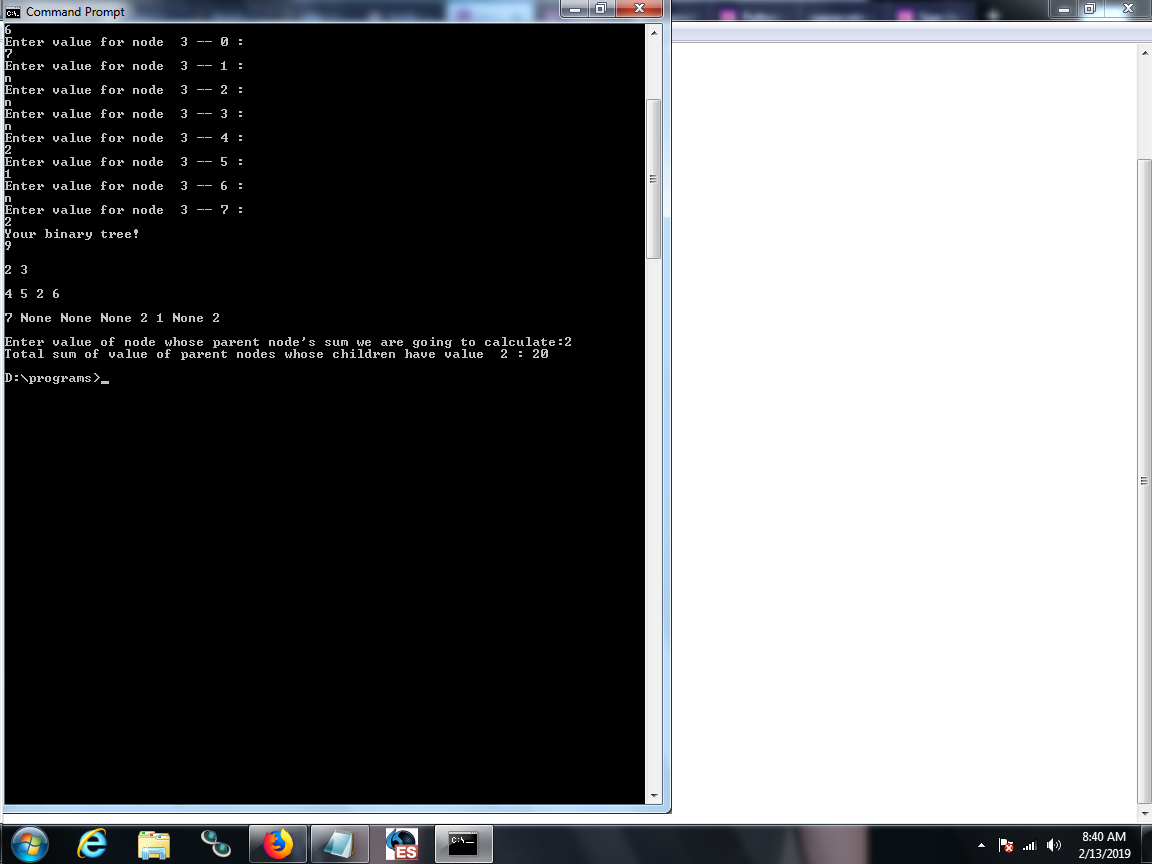## Code Syntax Highlighter

Preview of the formatted code :-
``````#22------	Python program to find sum of all the parent nodes having child node x	-------Arun Prakash B
#Enter the depth of tree with the root starting at level 0

d=int(input("Enter the depth of your binary tree:"))

#Initializing 2d array with value None to all nodes

t=[[None for j in range(2**(d-1))] for i in range(d)]

#User input to the nodes and None to empty nodes
print("Enter integer values to nodes and n to empty nodes:")
for i in range(d):

for j in range(2**i):
print("Enter value for node ", i,"--",j,": ")
temp=input()
if temp!="n":
t[i][j]=int(temp)

for i in range(d):

for j in range(2**i):
print(t[i][j], end=" ")

print("\n")

#Enter value of node whose parent node's sum we are going to calculate"

x=int(input("Enter value of node whose parent node's sum we are going to calculate:"))
sum=0
for i in range(d-2,-1,-1):
childNode=2**(i+1)-1
for j in range(2**i-1,-1,-1):
if t[i+1][childNode]==x:
sum+=t[i][j]
childNode-=1
if t[i+1][childNode]==x:
sum+=t[i][j]
childNode-=1

print("Total sum of value of parent nodes whose children have value ", x, ":", sum)``````#### More Articles of Arunprakash B:

Name Views Likes
Python program to find sum of all the parent nodes having child node x 117 12Input: